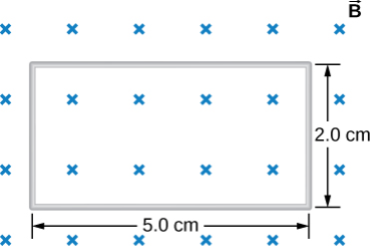# #

## Question Text#

The figure below shows a single-turn rectangular coil that has a resistance of $${{params.R }}\rm \ \Omega$$. The magnetic field at all points inside the coil varies according to $$B=B_0e^{-{\alpha}t}$$, where $$B_0$$ = $${{params.B }}\textrm{ T}$$ and $$\alpha$$ = $${{params.f }}\textrm{ Hz}$$. What is the current induced in the coil at $${{params.t }}\textrm{ s}$$?$$I=$$ $$\rm\ A$$